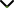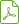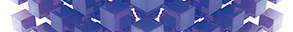# Mathematical Models for Supply Chain Management

Publishing date
11 Mar 2016
Status
Published
23 Oct 2015

Guest Editors

1Hanyang University, Seoul, Republic of Korea

2Poznan University of Technology, Poznan, Poland

3Soochow University, Taipei, Taiwan

# Mathematical Models for Supply Chain Management

## DescriptionBusiness leaders today are facing many decision problems including profit, cost, efficiency, responsiveness, quality, material availability, and new market entry to design and configure their supply chains continually. Also as supply chains become globalized the complexities to manage and control those globalized supply chains will also increase use of mathematical models for design and operation of their supply chains.

The attention towards the use of mathematical models to analyse and optimize supply chain has been increasing, mainly because of their lower cost and greater capability. In supply chain management the use of mathematical modelling is not specific to any particular level; they can be used at any level (strategic, tactical, or operational). Mathematical modelling approaches that are usually considered for supply chain decision problems include linear programming, mixed integer/integer linear programming, nonlinear programming, multiobjective programming, fuzzy mathematical programming, stochastic programming, heuristics algorithms, and metaheuristics and hybrid models.

The guest editors are interested in all types of rigorously developed supply chain mathematical models and their implementation for solving and analysing supply chain decision problems.

Potential topics include, but are not limited to:

• Mathematical analysis of operation strategies in SCM
• Optimization models for planning and operation of supply chain
• Mathematical models for financial SCM/supply chain finance
• Mathematical models for big data in SCM
• Simulation optimization approaches in SCM
• Mathematical models for logistics system in SCM
• Mathematical approaches for environmental issues/sustainability issues for SCM
• Mathematical models for supplier management and outsourcing in SCM
• Mathematical models for location and layout problems in SCM
• Mathematical models for supply chain risk management
• Mathematical approaches for humanitarian SCM
• Mathematical models for healthcare supply chain management
• Mathematical approaches for product safety in SCM
• Mathematical approaches for design and operation of robust and resilient supply chain
• Mathematical analysis of green and closed loop supply chain
• Mathematical models for transhipment problems in SCMDownload Special IssueJournal metrics
See full report
Acceptance rate48%
Submission to final decision55 days
Acceptance to publication25 days
CiteScore2.100
Journal Citation Indicator0.420
Impact Factor1.430Author guidelinesEditorial boardDatabases and indexing

Article of the Year Award: Outstanding research contributions of 2021, as selected by our Chief Editors. Read the winning articles.